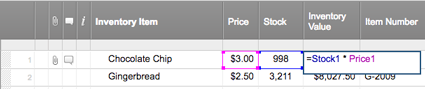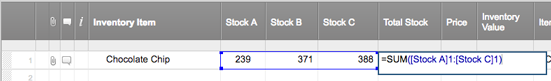# Create a Cell or Column Reference in a Formula

When you create formulas, it's possible to include values from other cells or columns on the sheet. You do this with cell or column references. You can create a reference to an individual cell, a range of cells, or an entire column.

It's also possible to reference data from other sheets. For information on referencing data from other sheets, see Formulas: Reference Data from Other Sheets.

### Summary of Formula Reference Types

Here’s a cheat sheet you can use as you build formulas of your own.

To reference this Format it this way Example
Individual cell Column name, row number =Budget1
Column name contains a space or ends in a number Enclose the column name in brackets. =[Column A]1
=DAY([Q1]1)
Absolute reference (always refers to that specific cell, row, or column) Type a \$ symbol in front of the column name, row number or both. =\$[Column A]\$1
=[Column B]\$1
=\$[Column C]1
Multiple, discontinuous cells Use a comma between cell references. =SUM(Budget1, Expenses4, [Projected Earnings]20)
A range of cells in the same column Reference the first cell in the range, then the last cell, separated by a :(colon). =SUM(Budget1:Budget12)
An entire column (including any newly added cells) The column name separated by a : (colon). =SUM(Budget:Budget)
A range of cells across multiple columns Reference the upper-rightmost cell, then the lower-leftmost cell, seperated by a : (colon). =SUM(January1:March5)
An individual cell, range of cells, or complete columns from another sheet Type the name of a previously created reference to another sheet, wrapped in curly braces. =COUNT({my_sheet1 Range1})

### More Detailed Description of Reference Types

#### Reference Individual Cells

While building your formula, you can select a cell to reference it and work with that cell’s data in your formula. (You can also manually type the column name and row number to reference the cell.)

For example, the formula in the Inventory Value column of the following inventory management sheet will multiply the value from row 1 of the Price column to the value in row 1 of the Stock column:The formula returns \$2,994.00, the total worth of that item:#### Reference Column Names that Have Spaces or End in Numbers

If a column name contains spaces or contains any special characters or numbers, you must enclose it in brackets to avoid ambiguity:

=[Annual Budget]1 + [Annual Budget]2

=[Q1]1 + [Q2]1

=[Risk/Issue]5 + [Contingency/Mitigation]5

#### Create an Absolute Reference

You may have situations, such as when referencing a table with the VLOOKUP function, where you need to prevent Smartsheet from automatically updating cell references when a formula is moved or copied. To do this, create an absolute cell reference. (You can find details on the VLOOKUP function in the Function Reference article.)

To create an absolute reference, type \$ (a dollar sign) in front of the column name or row number in the cell reference of your formula. For example, if you move or copy the following formula, the column names and row numbers will not change for the cell references:

=\$[Column A]\$1 * \$[Column B]\$1

The following formula has absolute references to only the row numbers. If you move or copy the formula, the column references will change respectively based on the formula’s new location:

=[Column A]\$1 * [Column B]\$1

The following formula will maintain absolute references to the columns. If you move or copy the formula, the row numbers will change respectively based on the formula’s new location:

=\$[Column A]1 * \$[Column B]1

#### Reference a Range of Cells in the Same Column

To reference a range of cells, type a : (colon) between the two cell references.

For example, the formula at the bottom of the Inventory Value column of the following inventory management sheet will sum the values from row 1 to row 6 in the same column:The formula returns \$40,763.75, the total of all inventory values:#### Reference a Whole Column

You can reference an entire column in a formula, including all populated cells in the column. For example, the following formula sums all values in the Annual Budget column and will remain updated as new rows are added to or removed from the column:

=SUM([Annual Budget]:[Annual Budget])

NOTE: If a formula is placed in the column it's referencing, it won't reference the cell that the formula is in. For example, if you were to place the example formula above in a column named Annual Budget, the SUM formula would sum all cells except for the cell containing the formula.

#### Reference a Range Across Multiple Columns

To reference a range of values in a row across multiple columns, reference the first and last column in the row.

For example, the formula in the Total Stock column of the following inventory management sheet will sum the values from the Stock A, Stock B, and Stock C columns on row 1:The formula returns 998, the total stock from the three locations: